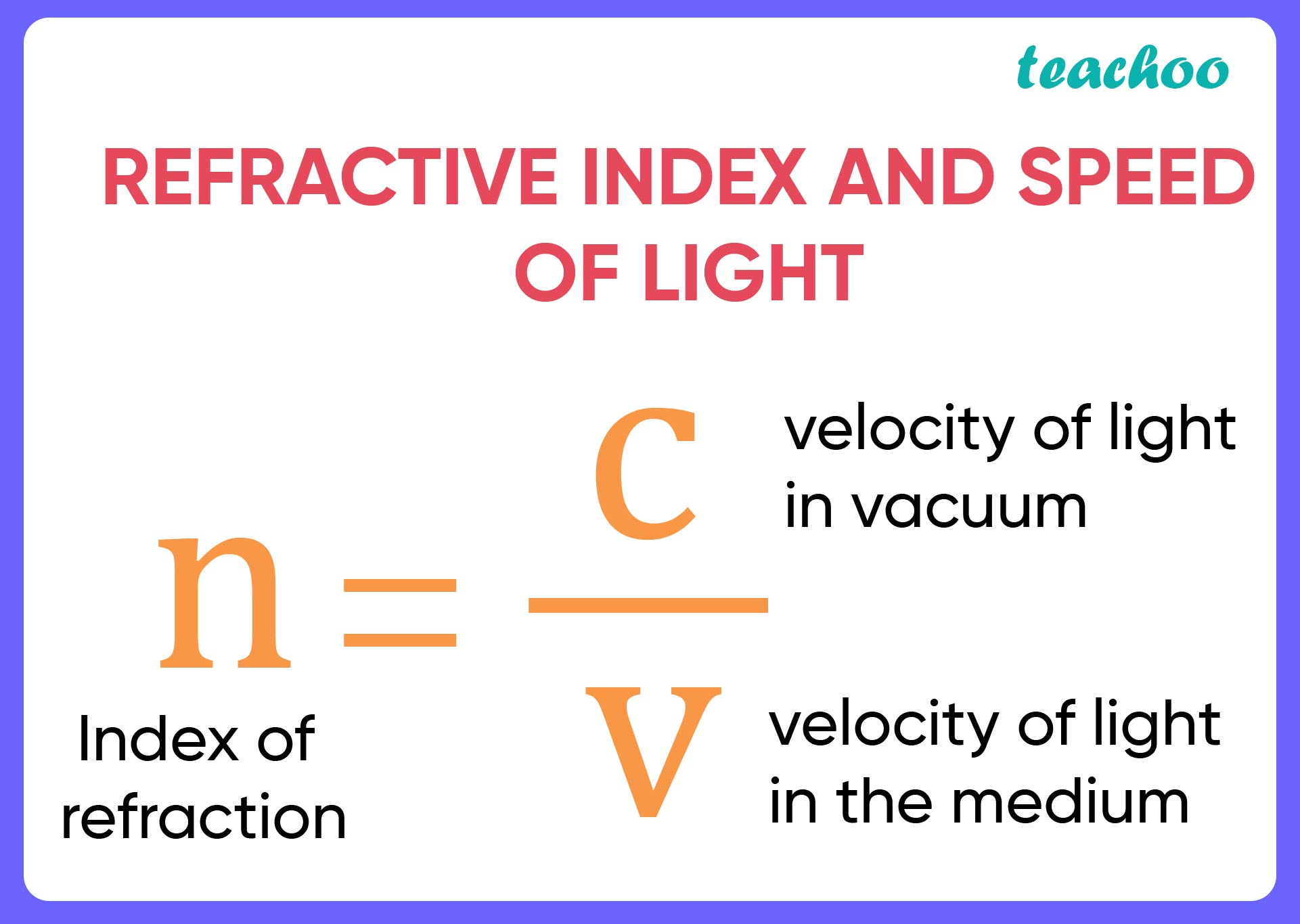Solutions - CBSE Class 10 Sample Paper for 2022 Boards - Science [MCQ]

Class 10
Solutions to CBSE Sample Paper - Science Class 10

## (D) iii and v are correct.

Let’s look at each options

• For (i) - A medium is rarer when the speed of light increases when light travels through that medium.
Since Speed of light in air is more than the speed of light in glass, which means air is optically rarer than glass. So, option (i) is incorrect.

• For (ii) - Actually, Speed of Light in Air is more than Speed of Light in Glass. Thus, they have different velocities. So, option (ii) is also incorrect .

• For (iii) - We discussed in part (i) that Air is optically rarer than glass. Thus, option (iii) is correct.

• For (iv) - Speed of light through a denser medium is slower than that of a rarer medium. So, option (iv) is also incorrect.• For (v) - The ratio of sin of angle of incidence in the first medium to the ratio of sin of angle of refraction in the second medium, gives the refractive index of the second material with respect to the first one. Thus, option (v) is correct.

Thus, options (iii) and (v) are correct.

So, the correct answer is (d)

Learn in your speed, with individual attention - Teachoo Maths 1-on-1 Class